回顾十年数据库流行度，哪款是你的最爱

+关注继续查看

数据获取

Method 1

https://db-engines.com/en/ranking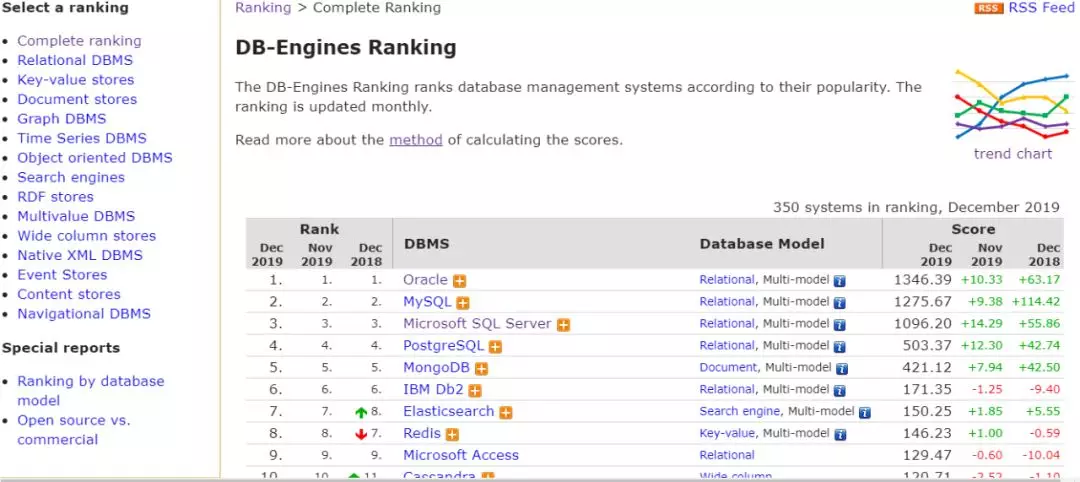https://db-engines.com/en/ranking_trend/system/Oracle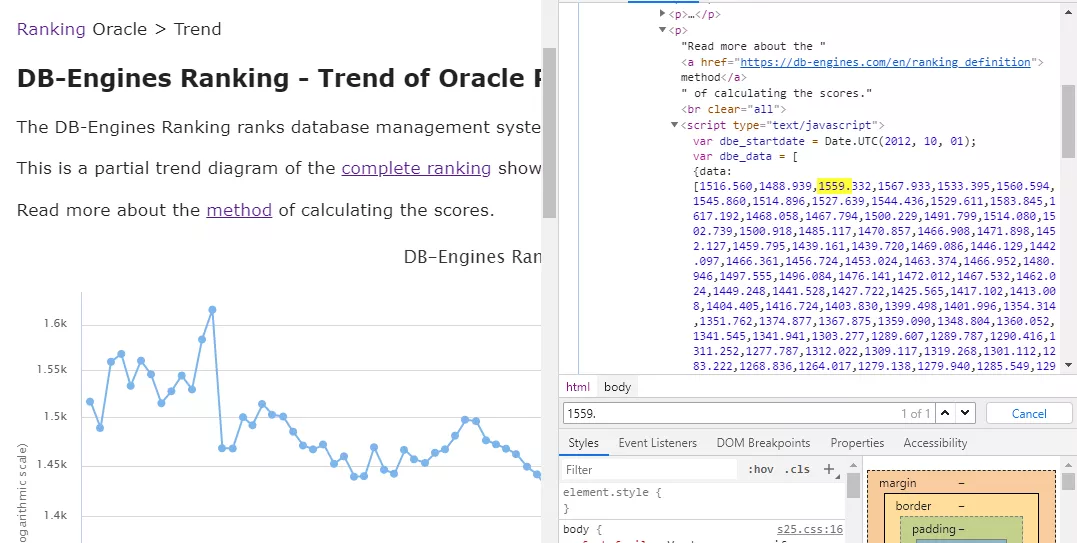import pandas as pd

mystr = ' Detailed vendor-provided information available'

def set_column3(column3):
if mystr in column3:
column3 = column3.split(mystr)
return column3

url = 'https://db-engines.com/en/ranking'
db_tb = tb.drop(index=[0, 1, 2])[[0, 1, 2, 3, 4, 5, 6, 7]]

# 处理数据
db_tb = db_tb.apply(set_column3)

# 保存数据
db_tb.to_csv('db_tb.csv')

async def fetch(session, url):
async with session.get(url) as response:

return await response.text()

async def get_db_data(db_name):
url = 'https://db-engines.com/en/ranking_trend/system/%s' % db_name

async with aiohttp.ClientSession() as session:
res = await fetch(session, url)
content = BeautifulSoup(res, "html.parser")
content.find_all("script")
db_data = content.find_all("script").string
src_text = js2xml.parse(db_data)
src_tree = js2xml.pretty_print(src_text)
data_tree = BeautifulSoup(src_tree, 'html.parser')
data_tree.find_all('number')
data = []
for i in data_tree.find_all('number'):
data.append(i['value'])

date_list = gen_time('%s-%s' % (data, str(int(data) + 1)))
date_value = list(zip(date_list, data[3:]))
d_data = zip([db_name for i in range(len(date_value))], date_value)

await save_data(d_data)

def gen_time(datestart, dateend=None):
if dateend is None:
dateend = time.strftime('%Y-%m', time.localtime(time.time()))
datestart=datetime.datetime.strptime(datestart, '%Y-%m')
dateend=datetime.datetime.strptime(dateend, '%Y-%m')
date_list = list(OrderedDict(((datestart + timedelta(_)).strftime(r"%Y-%m"), None) for _ in range((dateend - datestart).days)).keys())
date_list.append('2019-12')
return date_list

if __name__ == '__main__':
db_name = db_tb['3'].values.tolist()
loop = asyncio.get_event_loop()
tasks = [get_db_data(name) for name in db_name]
loop.close()

Method 2

https://db-engines.com/en/ranking_trend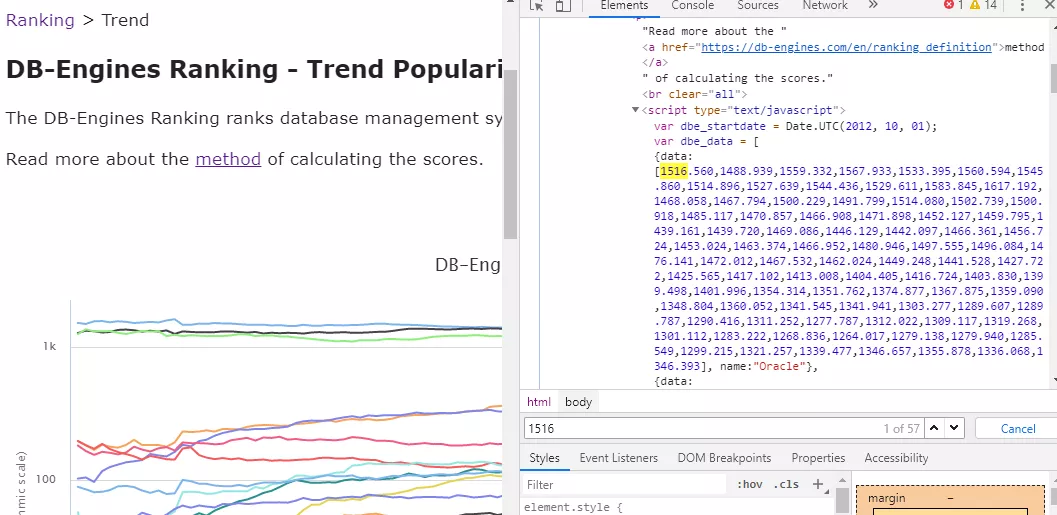for i in data_tree.find_all('object'):
date_list = gen_time('%s-%s' % (year_list, str(int(year_list) + 1)))
data = []
tmp_list = []
db_name = i.find('string')
if i.find('null'):
null_num = len(i.find_all('null'))
tmp_list = list(zip(date_list[:null_num], ['0' for i in range(null_num + 1)]))
date_list = date_list[null_num:]
for j in i.find_all('number'):
data.append(j['value'])

数据库总榜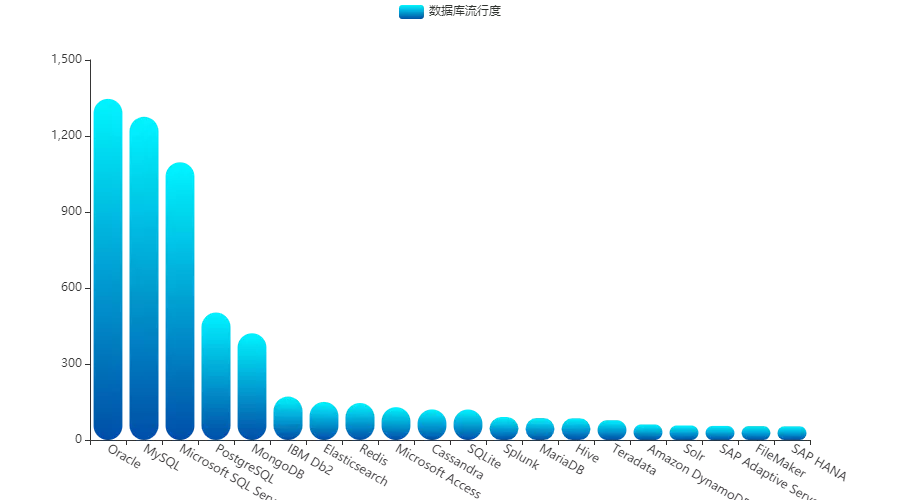MySQL 似乎从来没有令用户失望，也是稳稳的占据二哥的位置。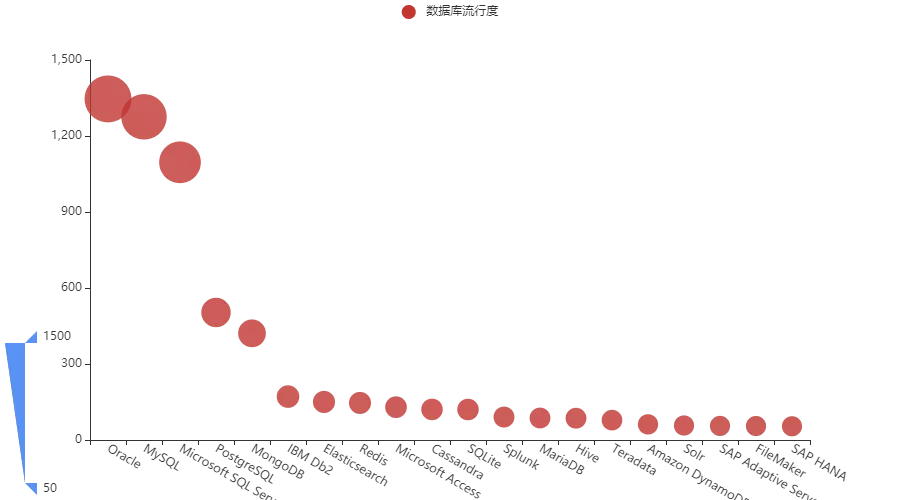主流数据库榜单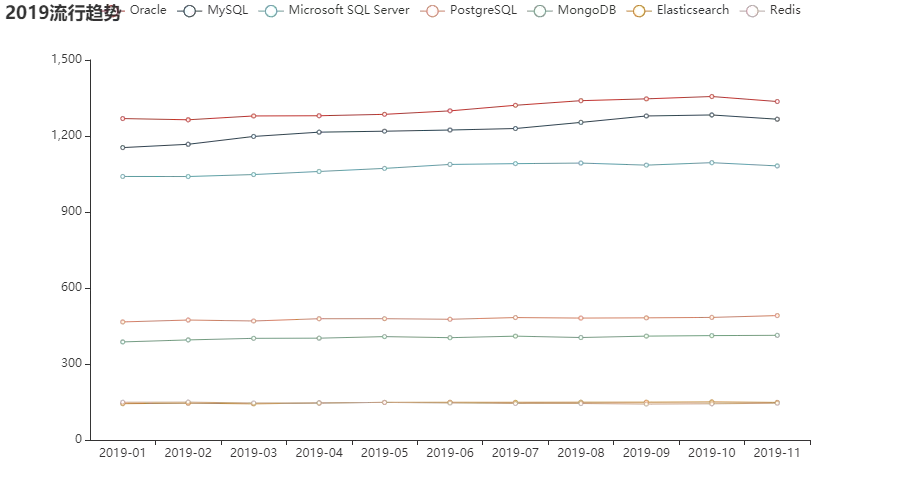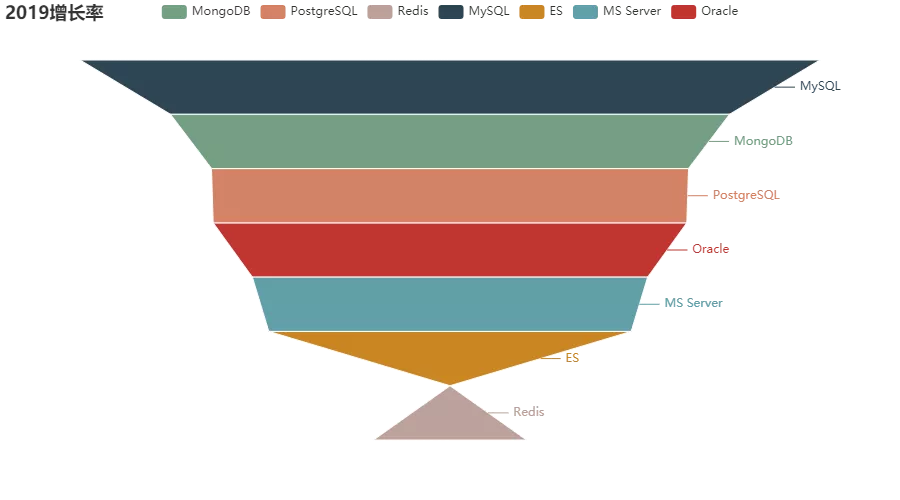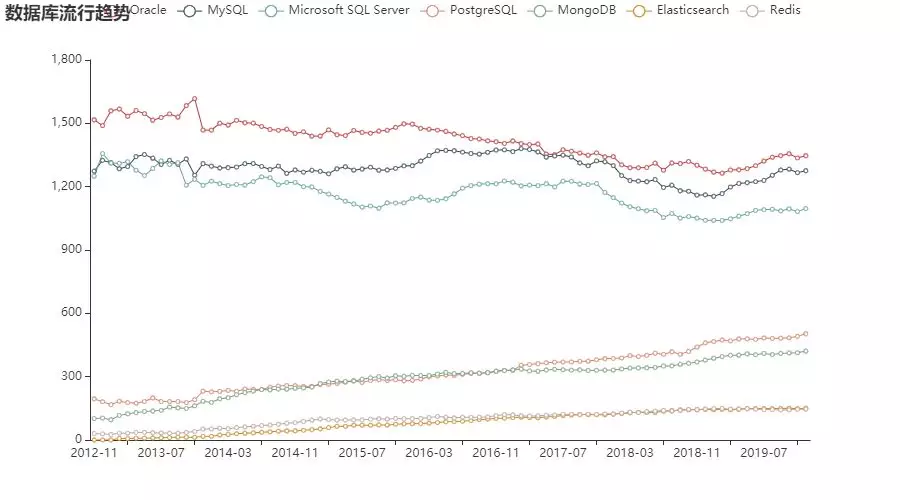Oracle 和 MS Server 整体来看确实呈现下降的趋势，而 MySQL 则稍稍有些增长。同时 PostgreSQL 增长比较明显，尤其是从 2017 年开始，流行度超越 MongoDB，相对应的，这个时间段也是榜首三大数据库的下滑期。

关系型数据库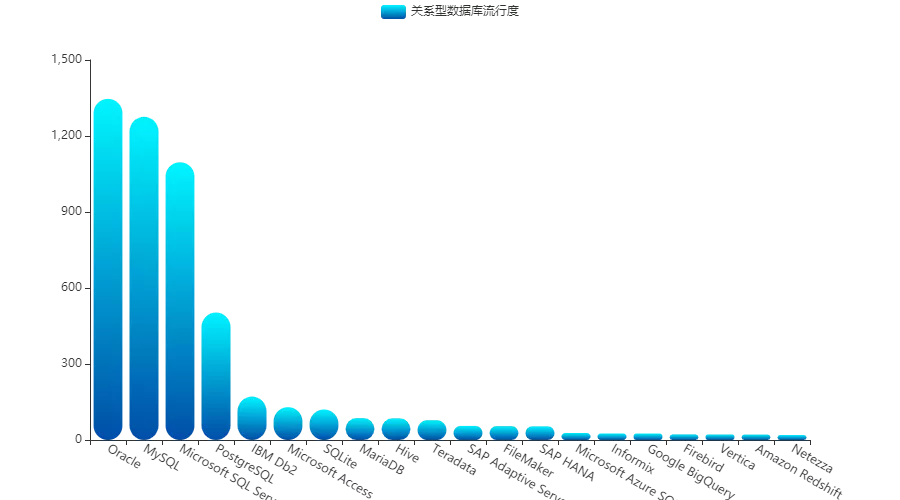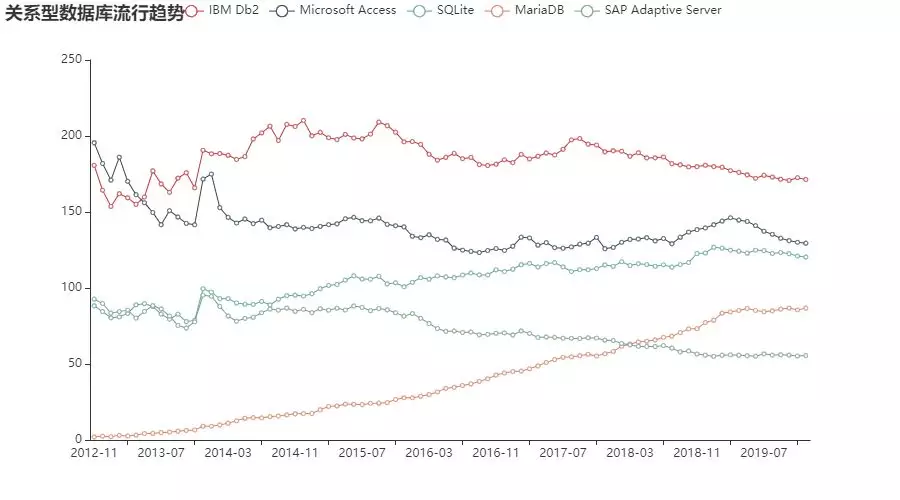key-value 数据库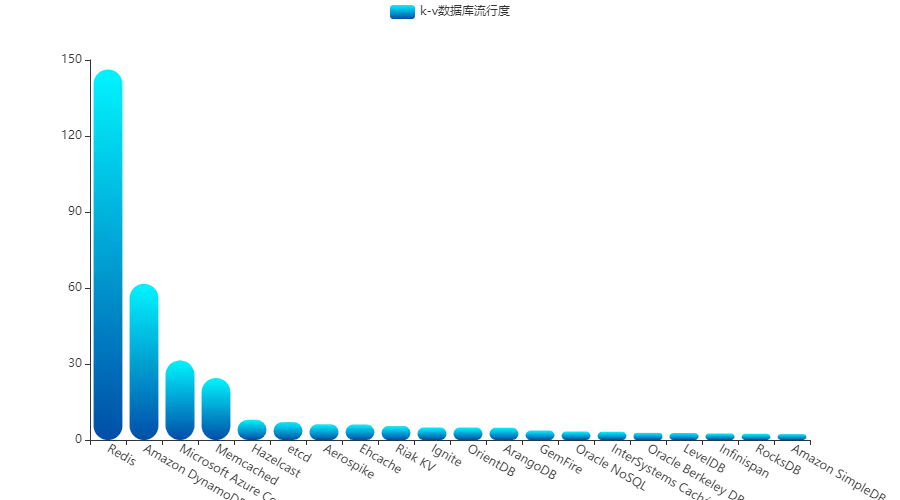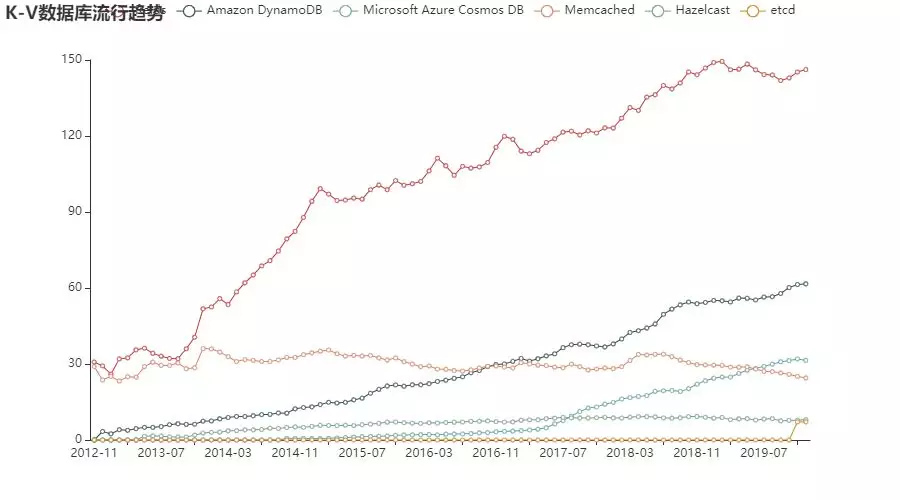文档数据库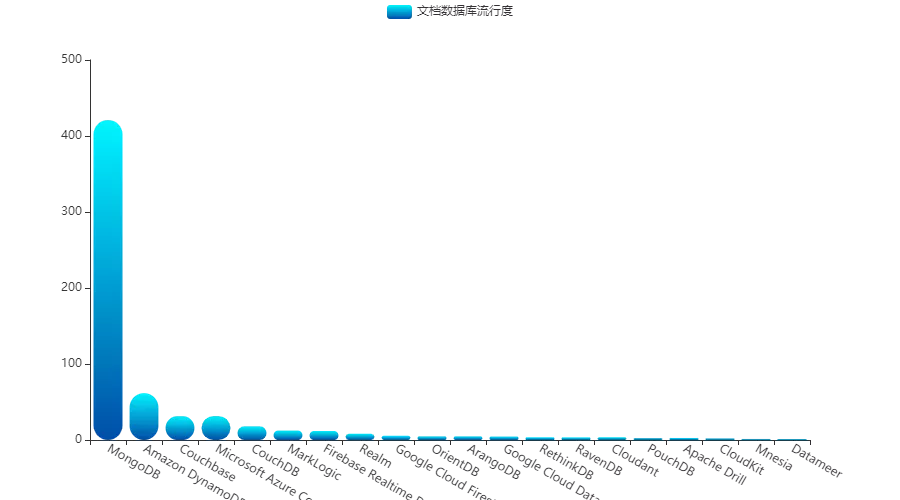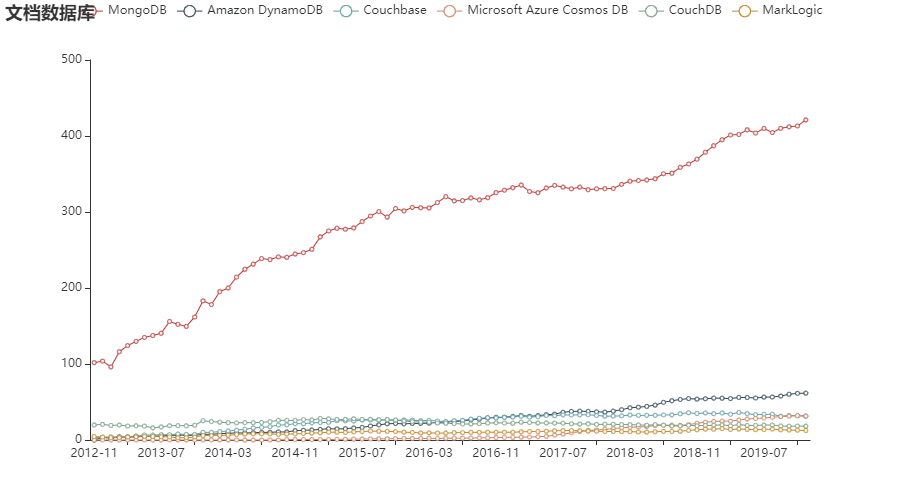时序数据库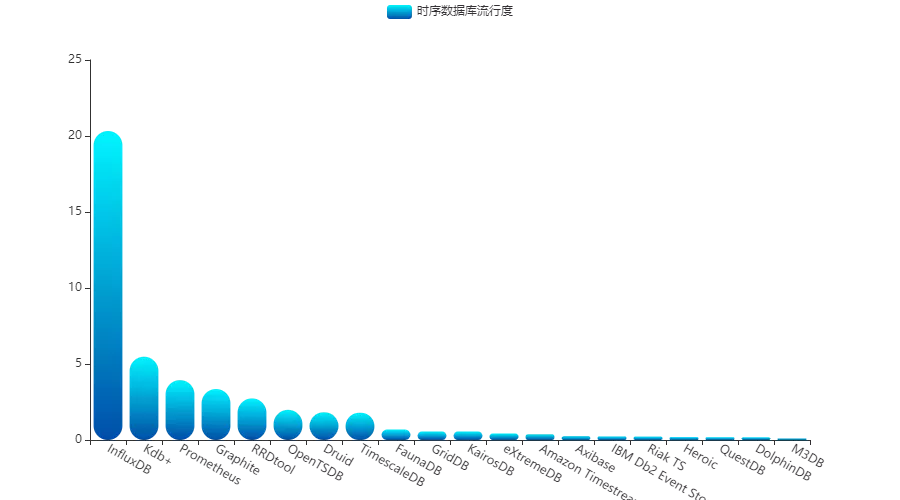Kdb、Prometheus 和 OpenTSDB 等都在各自擅长的领域发挥着不可替代的作用。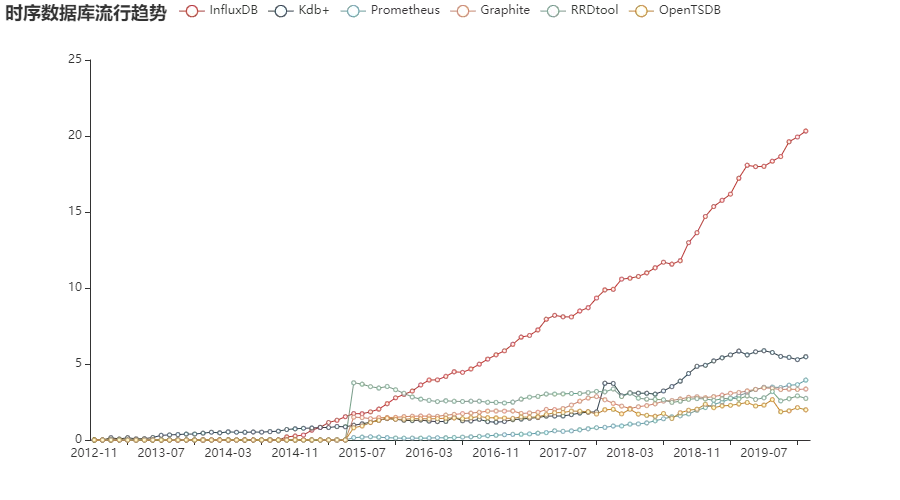图数据库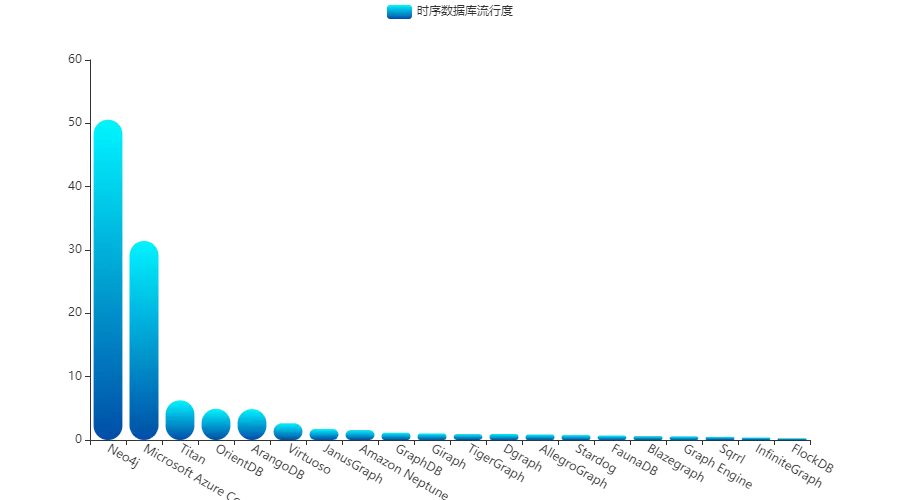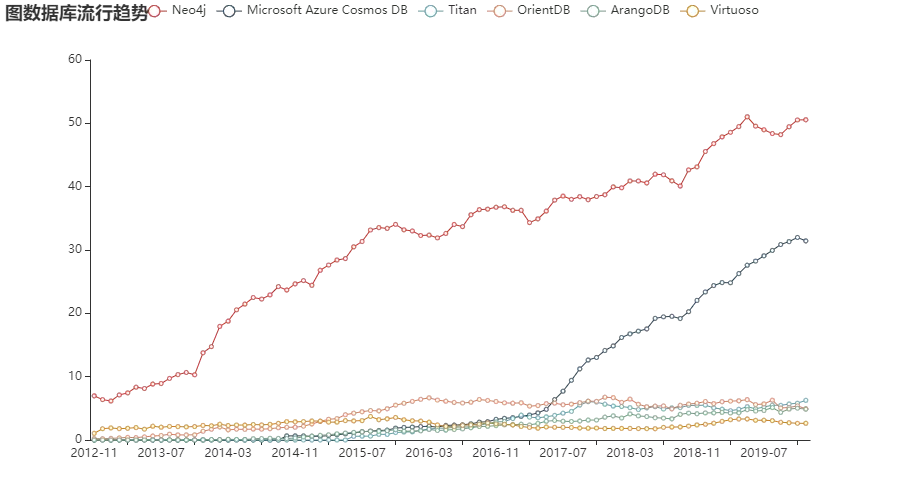Neo4j 和 Microsoft Azure Cosmos DB 走势迅猛，看来在知识图谱兴起的时代，图数据库也要呈现二分天下的态势了。

搜索数据库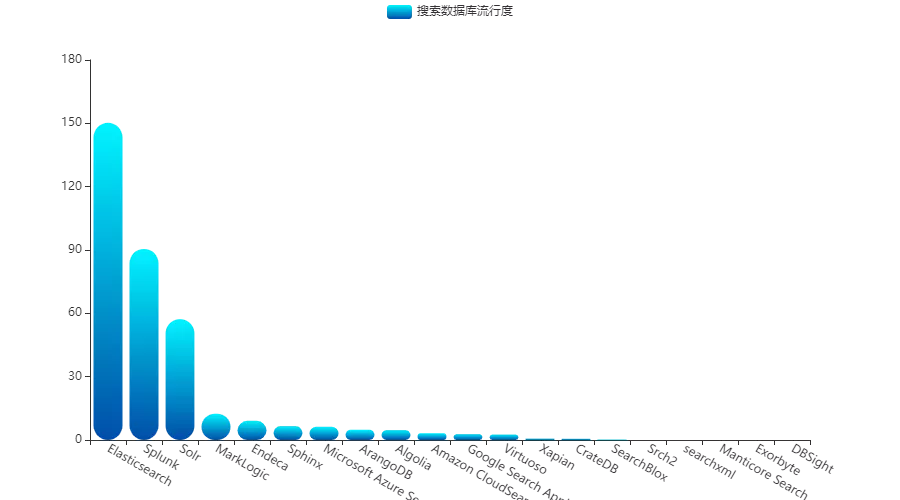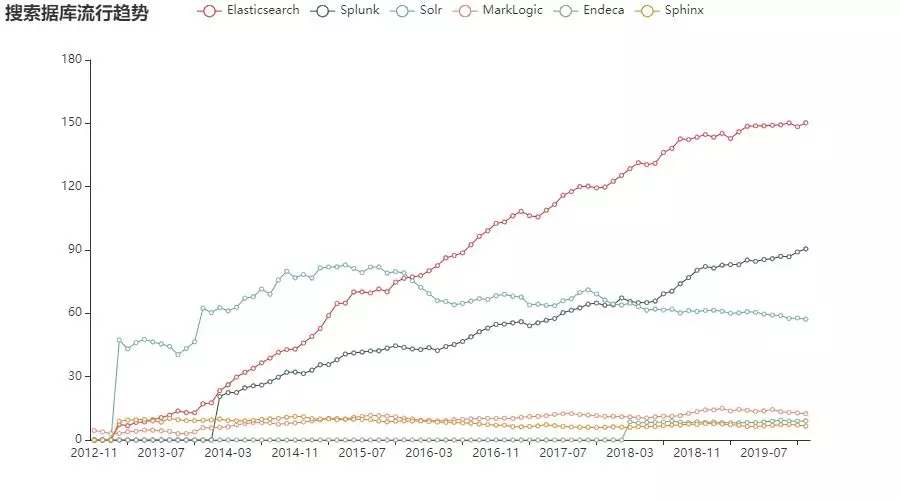1043 0thinkphp 3.2分布式数据库读写分离扩展阅读
5382 0AI(OtterTune)引波澜 - AI会洗牌数据库行业吗? DBA如何转变思想
2992 0752 01794 0Oracle :一次数据库连接，返回多个结果集
1233 01570 0【云栖神侠传】—阿里云数据库专家德歌告诉你PostgreSQL的那些事

4932 01052 0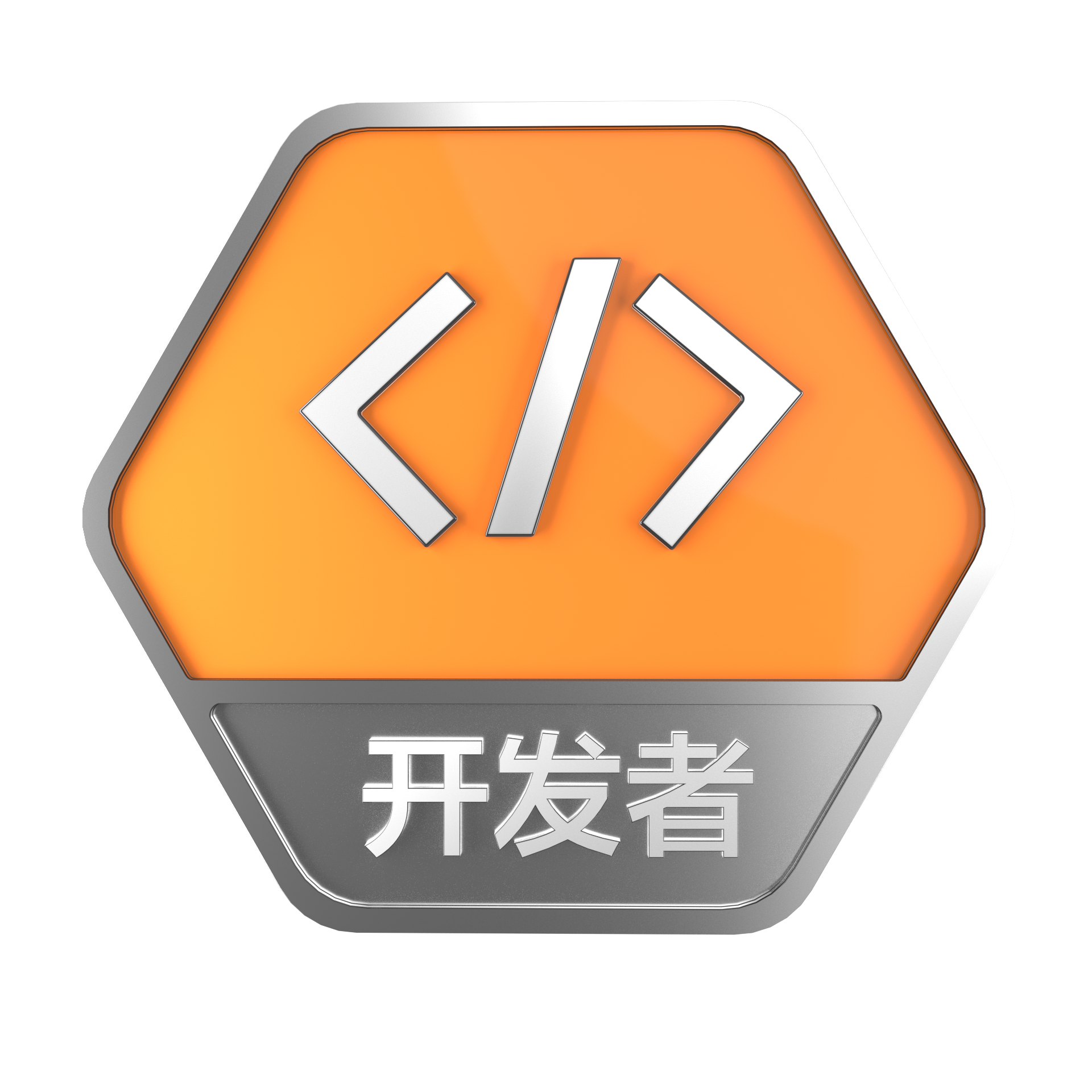+关注
204

1654

《2021云上架构与运维峰会演讲合集》

《零基础CSS入门教程》

《零基础HTML入门教程》